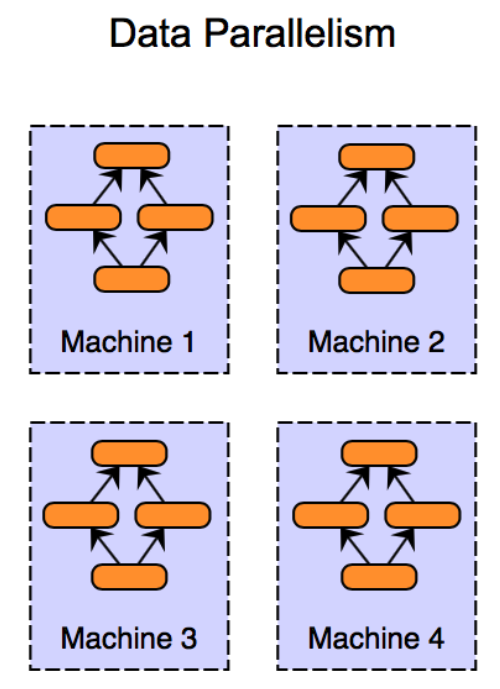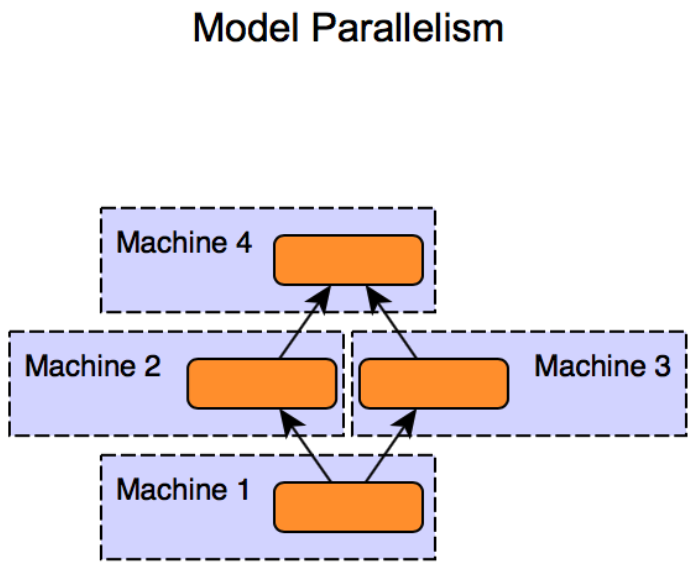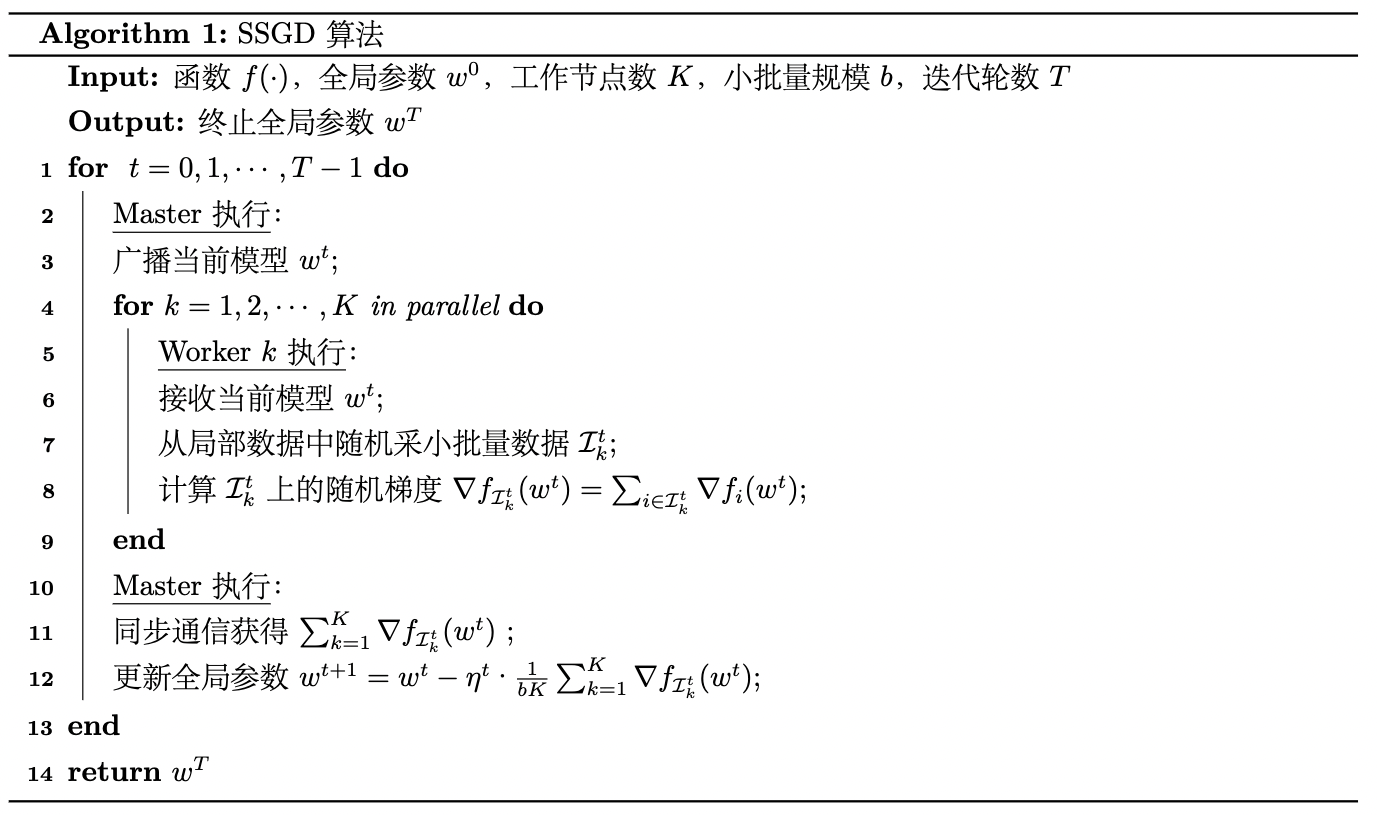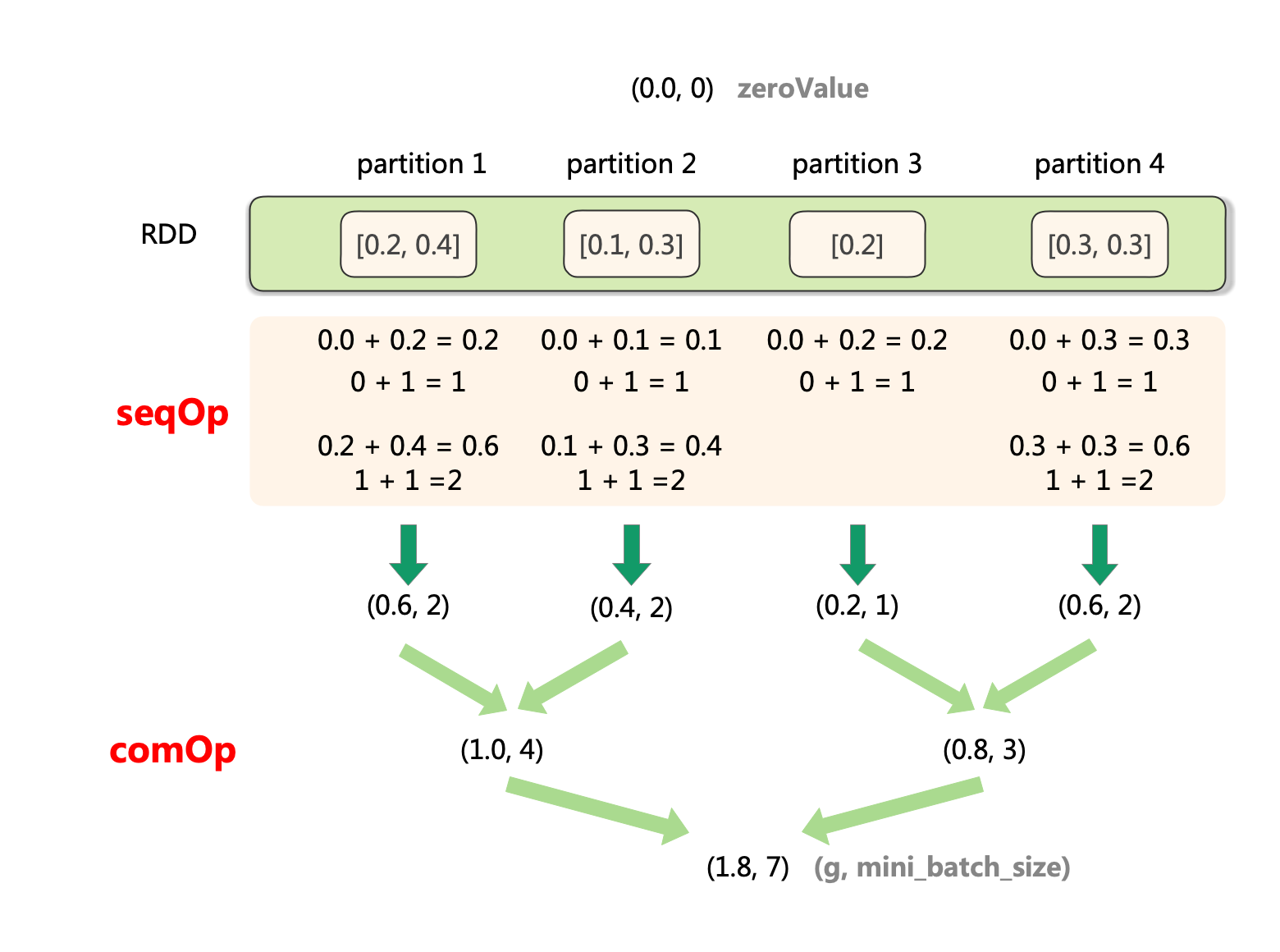<input id="ohw05"></input>
• <var id="ohw05"></var>
• <code id="ohw05"><cite id="ohw05"></cite></code>
<label id="ohw05"></label>
<var id="ohw05"></var>
• # 分布式機器學習：同步并行SGD算法的實現與復雜度分析（PySpark）

## 1 分布式機器學習概述

大規模機器學習訓練常面臨計算量大、訓練數據大（單機存不下）、模型規模大的問題，對此分布式機器學習是一個很好的解決方案。

1）對于計算量大的問題，分布式多機并行運算可以基本解決。不過需要與傳統HPC中的共享內存式的多線程并行運算（如OpenMP）以及CPU-GPU計算架構做區分，這兩種單機的計算模式我們一般稱為計算并行）。

2）對于訓練數據大的問題，需要將數據進行劃分并分配到多個工作節點(Worker)上進行訓練，這種技巧一般被稱為數據并行。每個工作節點會根據局部數據訓練出一個本地模型，并且會按照一定的規律和其他工作節點進行通信（通信內容主要是本地模型參數或者參數更新），以保證最終可以有效整合來自各個工作節點的訓練結果并得到全局的機器學習模型。如果是訓練數據的樣本量比較大，則需要對數據按照樣本進行劃分，我們稱之為“數據樣本劃分”，按實現方法可分為“隨機采樣法”和“置亂切分法”。樣本劃分的合理性在于機器學習中的經驗風險函數關于樣本是可分的，我們將每個子集上的局部梯度平均，仍可得到整個經驗風險函數的梯度。

如果訓練數據的維度較高，還可對數據按照維度進行劃分，我們稱之為“數據維度劃分”。它相較數據樣本劃分而言，與模型性質和優化方法的耦合度更高。如神經網絡中各維度高度耦合，就難以采用此方式。不過，決策樹對維度的處理相對獨立可分，將數據按維度劃分后，各節點可獨立計算局部維度子集中具有最優信息增益的維度，然后進行匯總。此外，在線性模型中，模型參數與數據維度是一一對應的，故數據維度劃分常與下面提到的模型并行相互結合。

3）對于模型規模大的問題，則需要對模型進行劃分，并且分配到不同的工作節點上進行訓練，這種技巧一般被稱為模型并行。與數據并行不同，模型并行的框架下各個子模型之間的依賴關系非常強，因為某個子模型的輸出可能是另外一個子模型的輸入，如果不進行中間計算結果的通信，則無法完成整個模型訓練。因此，一般而言，模型并行相比數據并行對通信的要求更高。這里提一下數據樣本劃分中的幾種劃分方式。給定$$n$$$$d$$維樣本和$$K$$個工作節點，數據樣本劃分需要完成的任務是將$$n$$個樣本以某種形式分配到$$K$$個工作節點上。

隨機采樣法中我們獨立同分布地從$$n$$個樣本中有放回隨機采樣，每抽取一個樣本將其分配到一個工作節點上。這個過程等價于先隨機采$$n$$個樣本，然后均等地劃分為$$K$$份。

隨機采樣法便于理論分析，但基于實現難度的考慮，在工程中更多采用的是基于置亂切分的劃分方法。即現將$$n$$個樣本隨機置亂，再把數據均等地切分為$$K$$份，再分配到$$K$$個節點上進行訓練。置亂切分相當于無放回采樣，每個樣本都會出現在某個工作節點上，每個工作節點的本地數據沒有重復，故訓練數據的信息量一般會更大。我們下面的劃分方式都默認采取置亂切分的方法。

我們在后面的博客中會依次介紹針對數據并行和模型并行設計的各種分布式算法。本篇文章我們先看數據并行中最常用的同步并行SGD算法（也稱SSGD）是如何在Spark平臺上實現的。

## 2 同步并行SGD算法描述與實現

SSGD對應的偽代碼可以表述如下：其中，SSGD算法每次依據來自$$K$$個不同的工作節點上的樣本的梯度來更新模型，設每個工作節點上的小批量大小為$$b$$，則該算法等價于批量大小為$$bK$$
的小批量隨機梯度下降法。

我們令$$f$$為邏輯回歸問題的正則化經驗風險。設$$w$$為權值(最后一維為偏置)，樣本總數為$$n$$$$\{(x_i, y_i)\}_{i=1}^n$$為訓練樣本集。樣本維度為$$d$$$$x_i\in \mathbb{R}^{d+1}$$（最后一維擴充），$$y_i\in\{0, 1\}$$。則$$f$$表示為：

$f(w) = \frac{1}{n} \sum_{i=1}^{n}\left[y_{i} \log \pi_{w}\left(x_{i}\right)+\left(1-y_{i}\right) \log \left(1-\pi_w\left(x_{i}\right)\right)\right] + \lambda R(w)$

這里

\begin{aligned} \pi_w(x) = p(y=1 \mid x; w) =\frac{1}{1+\exp \left(-w^{T} x\right)} \end{aligned}

其梯度表示如下：

$\nabla f{(w)} = -\sum_{i=1}^n(y_i - \frac{1}{\exp(-w^Tx)+1})x_i + \lambda\nabla R(w)$

這里正則項的梯度$$\nabla R(w)$$要分情況討論：

(1) 不使用正則化

此時顯然$$\nabla R(w)=0$$

(2) L2正則化($$\frac{1}{2}\lVert w\rVert^2_2$$)

此時$$\nabla R(w)=w$$

(3) L1正則化($$\lVert w \rVert_1$$)

該函數不是在每個電都對$$w$$可導，只來采用函數的次梯度(subgradient)來進行梯度下降。$$\lVert w \rVert_1$$的一個比較好的次梯度估計是$$\text{sign}(w)$$。相比于標準的梯度下降，次梯度下降法不能保證每一輪迭代都使目標函數變小，所以其收斂速度較慢。

(4) Elastic net正則化($$\alpha \lVert w \rVert_1 + (1-\alpha)\frac{1 }{2}\lVert w\rVert^2_2$$)

$$\lVert w\rVert_1$$使用次梯度計算式，$$\frac{1}{2}\lVert w\rVert_2^2$$使用其梯度計算式，得最終的梯度計算式為$$\alpha \text{sign}(w) + (1-\alpha) w$$

我們約定計算第$$K$$個節點小批量$$\mathcal{I_k}$$的經驗風險的梯度$$\nabla f_{\mathcal{I_k}}(x)$$時不包含正則項的梯度，最終將$$K$$個節點聚合后再加上正則項梯度。

用PySpark對上述算法實現如下：

from sklearn.datasets import load_breast_cancer
import numpy as np
from pyspark.sql import SparkSession
from sklearn.model_selection import train_test_split
from sklearn.metrics import accuracy_score

n_slices = 4  # Number of Slices
n_iterations = 300  # Number of iterations
eta = 10  # iteration step_size, because gradient sum is divided by minibatch size, it shoulder be larger
mini_batch_fraction = 0.1 # the fraction of mini batch sample
lam = 0.001 # coefficient of regular term

def logistic_f(x, w):
return 1 / (np.exp(-x.dot(w)) + 1)

""" Compute linear regression gradient for a matrix of data points
"""
y = point[-1]    # point label
x = point[:-1]   # point coordinate
# For each point (x, y), compute gradient function, then sum these up
# notice thet we need to compute minibatch size, so return(g, 1)
return - (y - logistic_f(x, w)) * x

"""
assert(reg_type in ["none", "l2", "l1", "elastic_net"])
if reg_type == "none":
return 0
elif reg_type == "l2":
return w
elif reg_type == "l1":
return np.sign(w)
else:
return alpha * np.sign(w) + (1 - alpha) * w

if __name__ == "__main__":

D = X.shape
X_train, X_test, y_train, y_test = train_test_split(
X, y, test_size=0.3, random_state=0, shuffle=True)
n_train, n_test = X_train.shape, X_test.shape

spark = SparkSession\
.builder\
.appName("SGD")\
.getOrCreate()

matrix = np.concatenate(
[X_train, np.ones((n_train, 1)), y_train.reshape(-1, 1)], axis=1)

points = spark.sparkContext.parallelize(matrix, n_slices).cache()

# Initialize w to a random value
w = 2 * np.random.ranf(size=D + 1) - 1
print("Initial w: " + str(w))

for t in range(n_iterations):
print("On iteration %d" % (t + 1))

(g, mini_batch_size) = points.sample(False, mini_batch_fraction, 42 + t)\
.treeAggregate(
(0.0, 0),\
seqOp=lambda res, g: (res + g, res + 1),\
combOp=lambda res_1, res_2: (res_1 + res_2, res_1 + res_2)
)

w -= eta * g/mini_batch_size + lam * reg_gradient(w, "l2")

y_pred = logistic_f(np.concatenate(
[X_test, np.ones((n_test, 1))], axis=1), w)
pred_label = np.where(y_pred < 0.5, 0, 1)
acc = accuracy_score(y_test, pred_label)
print("iterations: %d, accuracy: %f" % (t, acc))

print("Final w: %s " % w)
print("Final acc: %f" % acc)

spark.stop()


我們嘗試以$$L2$$正則化、0.001的正則系數運行。初始權重如下：

Initial w: [ 0.09802896  0.92943671 -0.04964225  0.63915174 -0.61839489  0.86300117
-0.04102299 -0.01428918  0.84966149  0.50712175  0.10373804 -0.00943291
0.47526645 -0.19537069 -0.17958274  0.67767599  0.24612002  0.55646197
-0.76646105  0.86061735  0.48894574  0.87838804  0.05519216 -0.14911865
0.78695568  0.26498925  0.5789493  -0.20118555 -0.79919906 -0.79261251
-0.77243226]


最終的模型權重與在測試集上的準確率結果如下：

Final w: [ 2.22381079e+03  4.00830646e+03  1.34874211e+04  1.38842558e+04
2.19902064e+01  5.08904164e+00 -1.79005399e+01 -8.85669497e+00
4.28643902e+01  1.74744234e+01  2.24167323e+00  2.89804554e+02
-1.05612399e+01 -5.93151080e+03  1.60754311e+00  2.92290287e+00
2.46318238e+00  1.51092034e+00  4.23645852e+00  1.38371670e+00
2.20694252e+03  5.18743708e+03  1.32612364e+04 -1.39388946e+04
3.03078787e+01  4.41094696e+00 -2.24172172e+01 -5.27976054e+00
6.10623037e+01  1.83347648e+01  2.78974813e+02]
Final acc: 0.912281


代碼中有兩個關鍵點，一個是points.sample(False, mini_batch_fraction, 42 + t)。函數sample負責返回當前RDD的一個隨機采樣子集（包含所有分區），其原型為：

RDD.sample(withReplacement: bool, fraction: float, seed: Optional[int] = None) → pyspark.rdd.RDD[T]


參數withReplacement的值為True表示采樣是有放回(with Replacement, 即replace when sampled out)，為False則表示無放回 (without Replacement)。如果是有放回，參數fraction表示每個樣本的期望被采次數，fraction必須要滿足$$\geqslant0$$；如果是無放回，參數fraction表示每個樣本被采的概率，fraction必須要滿足位于$$[0, 1]$$區間內。

還有一個關鍵點是

.treeAggregate(
(0.0, 0),\
seqOp=lambda res, g: (res + g, res + 1),\
combOp=lambda res_1, res_2: (res_1 + res_2, res_1 + res_2)
)


該函數負責對RDD中的元素進行樹形聚合，它在數據量很大時比reduce更高效。該函數的原型為

RDD.treeAggregate(zeroValue: U, seqOp: Callable[[U, T], U], combOp: Callable[[U, U], U], depth: int = 2) → U[source]


其中zeroValue為聚合結果的初始值，seqOp函數用于定義單分區(partition)做聚合操作的方法，該方法第一個參數為聚合結果，第二個參數為分區中的數據變量，返回更新后的聚合結果。combOp定義對分區之間做聚合的方法，該方法第一個參數為第二個參數都為聚合結果，返回累加后的聚合結果。depth為聚合樹的深度。

我們這里treeAggregate想要聚合得到一個元組(g, mini_batch_size)g為所有節點樣本的隨機梯度和，mini_batch_size為所有節點所采的小批量樣本之和，故我們將聚合結果的初始值zeroVlue初始化為(0,0, 0)。具體的聚合過程描述如下：

1. 對每個partition：
a. 初始化聚合結果為(0.0, 0)
b. 對當前partition的序列元素，依次執行聚合操作seqOp
c. 得到當前partition的聚合結果(partition_sum, partition_count)

2. 對所有partition：
a. 按照樹行模式合并各partition的聚合結果，合并方法為combOp
b. 得到合并結果(total_sum, total_count)

形象化地表示該聚合過程如下圖所示：## 3 算法收斂性及復雜度分析

### 3.1 收斂性和計算復雜度

我們在博客《數值優化：經典隨機優化算法及其收斂性與復雜度分析》中說過，假設目標函數$$f: \mathbb{R}^d\rightarrow \mathbb{R}$$$$\alpha$$-強凸函數，并且$$\beta$$光滑，如果隨機梯度的二階矩有上界，即$$\mathbb{E}_{i^t}{\lVert\nabla f_{i^t}(w^t) \rVert^2\leqslant G^2}$$，當步長$$\eta^t = \frac{1}{\alpha t}$$時，對于給定的迭代步數$$T$$，SGD具有$$\mathcal{O}(\frac{1}{T})$$的次線性收斂速率：

$\mathbb{E}[ f(w^T) - f(w^*) ] \leqslant \frac{2\beta G^2}{\alpha^2T}$

而這意味著SGD的迭代次數復雜度為$$\mathcal{O}(\frac{1}{\varepsilon})$$，也即$$\mathcal{O}(\frac{1}{\varepsilon})$$輪迭代后會取得$$\varepsilon$$的精度。

盡管梯度的計算可以被分攤到個計算節點上，然而梯度下降的迭代是串行的。每輪迭代中，Spark會執行同步屏障(synchronization barrier)來確保在各worker開始下一輪迭代前$$w$$已被更新完畢。如果存在掉隊者(stragglers)，其它worker就會空閑(idle)等待，直到下一輪迭代。故相比梯度的計算，其迭代計算的“深度”(depth)是其計算瓶頸。

### 3.2 通信復雜度

$2\text{log}_2(p)(L + \frac{m}{B})$
這里$$p$$為除去driver節點的運算節點個數，$$L$$是節點之間的通信延遲。$$B$$是節點之間的通信帶寬。$$M$$是每輪通信中節點間傳輸的信息大小。故消息能夠夠以$$\mathcal{O}(\text{log}p)$$的通信輪數在所有節點間傳遞。